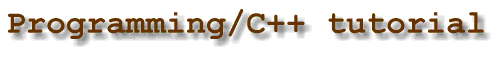Home | C++ Tutorial | 3. First Program | 1. A simple C++ program 2. A slightly more complex C++ program 3. Getting OrganizedShare This Page### A slightly more complex C++ program

Let's use the code below to find out what makes up a more complex C++ program -- one that uses a looping structure and some basic math operations. Adjust your browser so you can see the text window below the code. Then point your mouse at different statements in the program.
```#include <iostream>
#include <cmath>

using namespace std;

int main()
{
int x = 0;
while(x < 10) {
double y = sqrt((double)x);
cout << "The square root of " << x << " is " << y << endl;
x++;
}
return 0;
}
```

You may wish to copy this program into your C++ programming editor and compile it, just for practice. Because this is a special display program, it is somewhat more tricky to copy than others in this tutorial. And if you are using Arachnophilia, be sure to use the new edit menu function "Paste Text."

Note: You may notice I use the following line in most programs in this tutorial:
```using namespace std;
```
This line disables the C++ namespace feature. Namespaces are an important feature of C++, but for tutorial purposes I have decided the clarity of the program listings is more important. Later on, the topic of namespaces will be discussed.

### Discussion

This program's purpose is to repeat a statement sequence 10 times. The statement sequence, grouped by braces, prints a number and its square root. The program should execute the statement sequence repeatedly, beginning with the numeric value 0 and ending with the numeric value 9. Here are the steps to accomplish this goal:
1. Include a header file that contains many useful C++ things:
```#include <iostream>
```
2. Include another header file that contains the definitions of many useful math functions:
```#include <cmath>
```
Note: On some Unix systems, in order to use math functions, you need to explicitly tell the compiler about it, like this: "g++ programname.cpp -lm"

3. Disable the C++ namespace feature. Later on, in more complex programs, you will no longer want to do this:
```using namespace std;
```
4. Begin to define the standard function main(), and open its statement group:
```int main()
{
```
5. Define (create) an index variable, meant to contain the number whose square root is taken:
```int x = 0;
```
6. Create a loop control statement that tests for the desired end condition, and open its statement group:
```while(x < 10) {
```
7. Define a floating-point variable and, using a member of the math library (found in header file "cmath"), compute the square root of x:
```double y = sqrt((double)x);
```
Q: Why does variable y, the one that contains the square root, need to be a floating-point variable?
A: Because many square roots have fractional parts, which cannot be represented in integer variables. The square root of 2 is 1.41421356237, not 1.
Q: Why must the index variable x be converted to a double (in the statement "(double)x") before being submitted to the sqrt() function?
A: Because none of the available "versions" of the sqrt() function accepts an integer. Try compiling this program without the conversion step and see what messages the compiler prints.

8. Print the result of the computation on the standard console output stream (cout):
```cout << "The square root of " << x << " is " << y << endl;
```
9. Increase the index variable by 1:
```x++;
```
10. Close the "while" statement group:
```}
```
11. Return an integer value to the calling process:
```return 0;
```
12. Close the "main" statement group:
```}
```

On first sight this may seem like an excessive focus on detail, but you will soon discover that successful programming relies on (among many things) the ability to pay attention to many seemingly small details. In fact, it is not an exaggeration to say that every single character in this program needs to be present, in the correct order, in the right case, spelled correctly, for the program to work at all. And this program only has a few lines. Now imagine the concentration required to make a 100,000-line program work.

But this impression is not entirely accurate. In modern programming, the program's various tasks can (and should) be encapsulated in modules. Each module can be compiled and tested separately, then, after they have been proven to work individually, they can be joined into a complete program. Without this crucial idea, included in something called "Object-Oriented Programming" (OOP), large, complex programs would not be possible.

 Home | C++ Tutorial | 3. First Program | 1. A simple C++ program 2. A slightly more complex C++ program 3. Getting OrganizedShare This Page